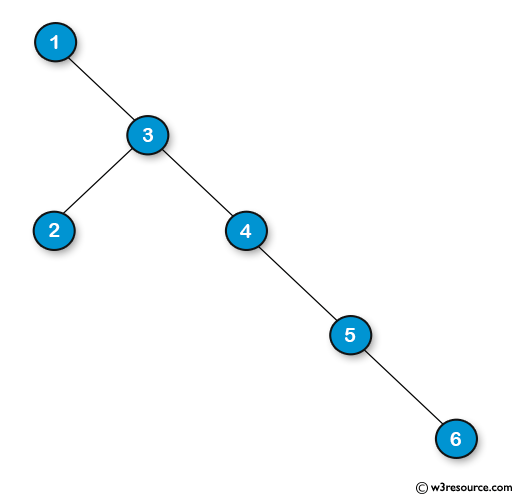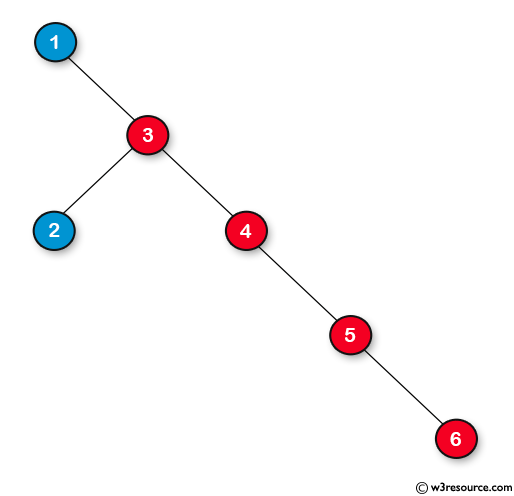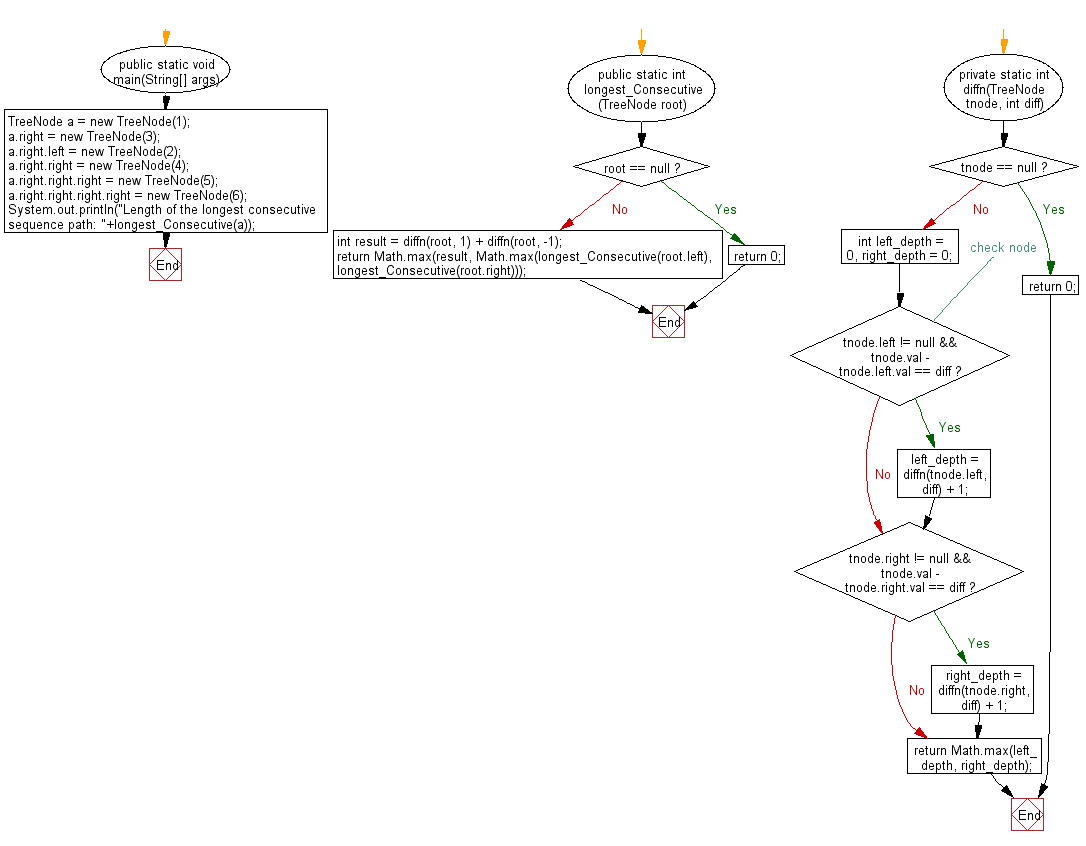﻿ Java programming exercises: Find the length of the longest consecutive sequence path of a given binary tree - w3resource# Java Exercises: Find the length of the longest consecutive sequence path of a given binary tree

## Java Basic: Exercise-184 with Solution

Write a Java program to find the length of the longest consecutive sequence path of a given binary tree.

Note: The longest consecutive path need to be from parent to child.

Sample Binary tree:Result:Sample Solution:

Java Code:

``````import java.util.*;
public class Solution {
public static void main(String[] args) {
TreeNode a = new TreeNode(1);
a.right = new TreeNode(3);
a.right.left = new TreeNode(2);
a.right.right = new TreeNode(4);
a.right.right.right = new TreeNode(5);
a.right.right.right.right = new TreeNode(6);
System.out.println("Length of the longest consecutive sequence path: "+longest_Consecutive(a));
}
public static int longest_Consecutive(TreeNode root) {
if (root == null) {
return 0;
}
int result = diffn(root, 1) + diffn(root, -1);
return Math.max(result, Math.max(longest_Consecutive(root.left), longest_Consecutive(root.right)));
}
private static int diffn(TreeNode tnode, int diff) {
if (tnode == null) {
return 0;
}
int left_depth = 0, right_depth = 0;
// check node
if (tnode.left != null && tnode.val - tnode.left.val == diff) {
left_depth = diffn(tnode.left, diff) + 1;
}
if (tnode.right != null && tnode.val - tnode.right.val == diff) {
right_depth = diffn(tnode.right, diff) + 1;
}
return Math.max(left_depth, right_depth);
}
}
```
```

Sample Output:

```Length of the longest consecutive sequence path:  4
```

Flowchart:Java Code Editor:

Company:

What is the difficulty level of this exercise?

﻿

## Java: Tips of the Day

Directory Content:

Java allows you to get the names of all subdirectories and files in a folder as an array, which can then be sequentially viewed.

```import java.io.*;

public class ListContents {
public static void main(String[] args) {
File file = new File("//home//user//Documents/");
String[] files = file.list();

System.out.println("Listing contents of " + file.getPath());
for(int i=0 ; i < files.length ; i++)
{
System.out.println(files[i]);
}
}
}
```